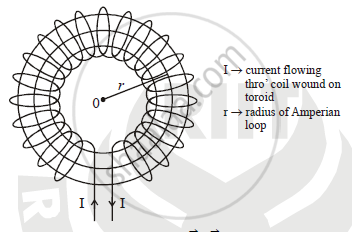HSC Science (Electronics) 12th Board ExamMaharashtra State Board
Share

Books Shortlist
Your shortlist is empty

# Obtain an Expression for Magnetic Induction Along the Axis of Toroid. - HSC Science (Electronics) 12th Board Exam - Physics

#### Question

Obtain an expression for magnetic induction along the axis of the toroid.

#### Solution

Magnetic induction along the axis of toroid:

The toroid is a solenoid bent into a shape of the hollow doughnut.

Consider a toroidal solenoid of average radius ‘r’ having center carrying the current I. In order to find magnetic field produced at the center along the axis of toroid due to the current flowing through the coil, imagine an Amperial loop of radius ‘r’ and traverse it in the clockwise direction.According to Ampere’s circuital law,

ointvecB.vec(dL)=mu_0I

Here current I flow through the ring as many times time as there are the number of turns. Thus the total current flowing through toroid is N I , where N is the total number of turns.

:.ointvecB.vec(dL)=mu_0NI" ---------(1)"

Now, and are in same direction :.ointvecB.vec(dL)=BointdL

:.ointvecB.vec(dL)=B(2pir)" ------(2)"

Comparing equation (1) and equation (2 )

μ0NI=B(2πr)  :.B=(mu_0NI)/(2pir) .......(3)

If ‘n’ is the number of turns per unit length of toroid then n=N/(2pir)

Substituting this value in equation No (3) we get B = μ0 n I

Is there an error in this question or solution?

#### APPEARS IN

2013-2014 (March) (with solutions)
Question 6.1 | 3.00 marks
2016-2017 (July) (with solutions)
Question 6.2 | 3.00 marks

#### Video TutorialsVIEW ALL 

Solution Obtain an Expression for Magnetic Induction Along the Axis of Toroid. Concept: Ampere’s Circuital Law.
S